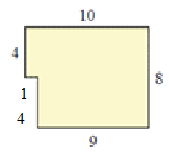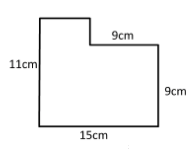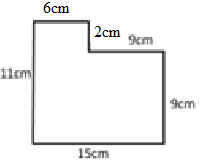# Finding the missing length in a figure

#### Complete Python Prime Pack

9 Courses     2 eBooks

#### Artificial Intelligence & Machine Learning Prime Pack

6 Courses     1 eBooks

#### Java Prime Pack

9 Courses     2 eBooks

In this lesson, we solve for missing lengths in given piecewise rectangular figures.

We are given piecewise rectangular figures with some measurements while some other ones are missing. We are required to find these missing lengths. In these figures, it is assumed that all intersecting sides meet in right angles. It is also noted that opposite sides of a rectangle are parallel and equal in length while calculating the missing lengths. We also split the given figure into two rectangles for same purpose.

Find the missing length(s) in the following figure.### Solution

Step 1:

Missing length = 8 – 4 = 4 units

Missing length = 10 – 9 = 1 unit

Step 2:

Figure with missing lengths shownFind the missing length(s) in the following figure.### Solution

Step 1:

Missing length = 15 – 9 = 6 cm

Missing length = 11 – 9 = 2 cm

Step 2:

Figure with missing lengths shown